Instant Maths Help:

# Edexcel A Level - Trapezium Rule

## Integration as Limit of Sum & Trapezium Rule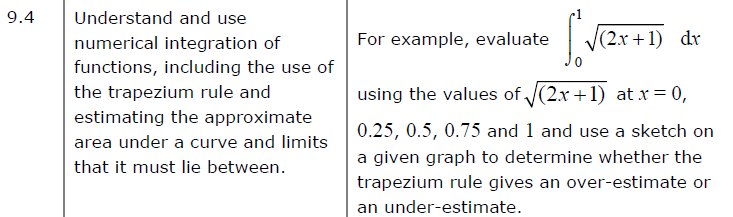##### Edexcel 9MA0/01 Jun 2023 A2 Exam Q. 5 :   6 marks in 7:12 min.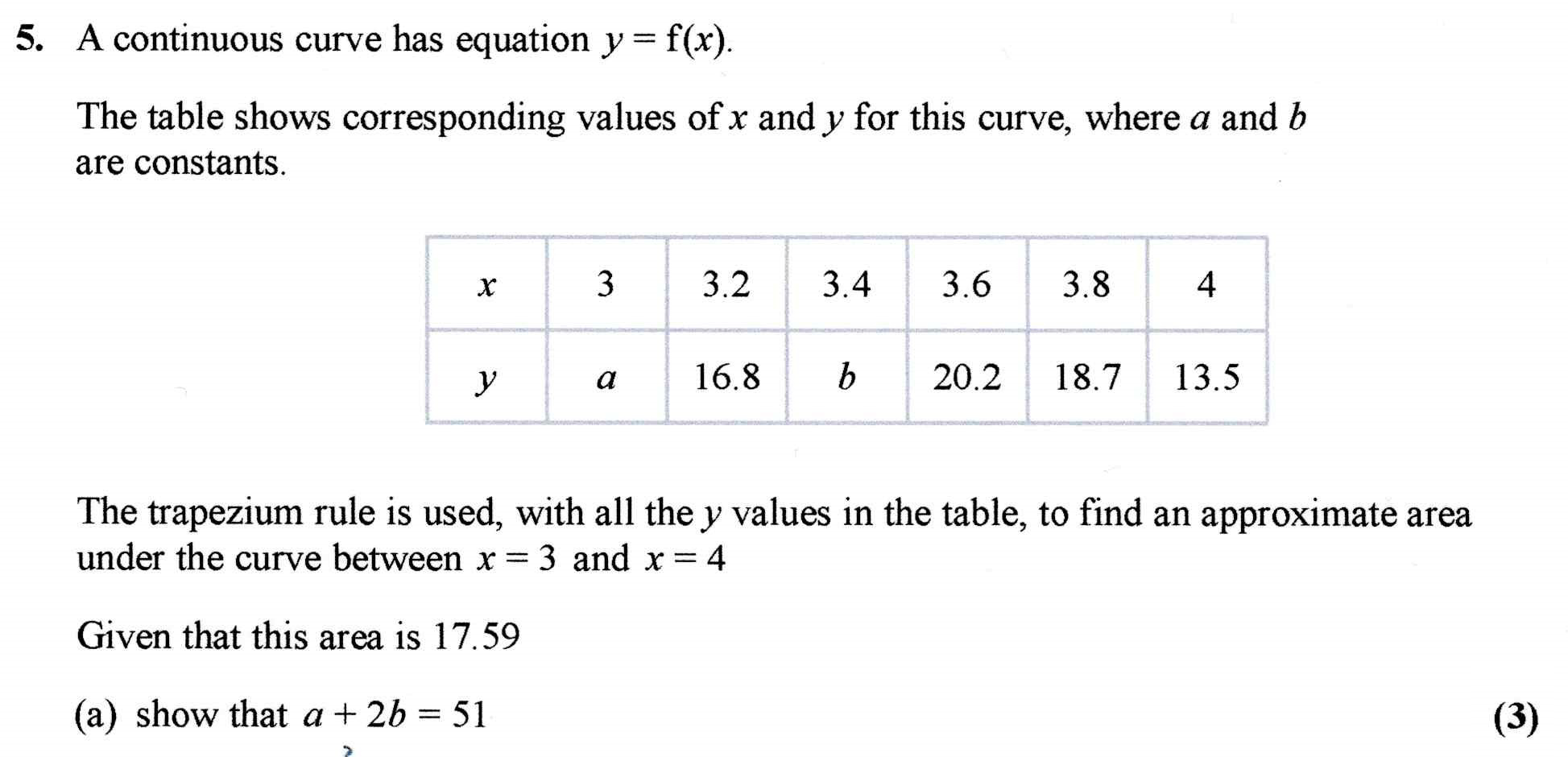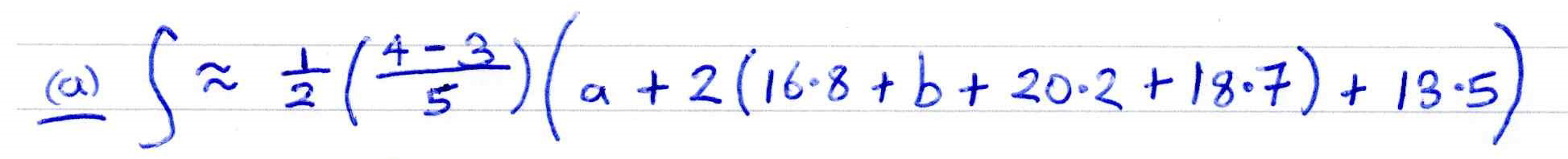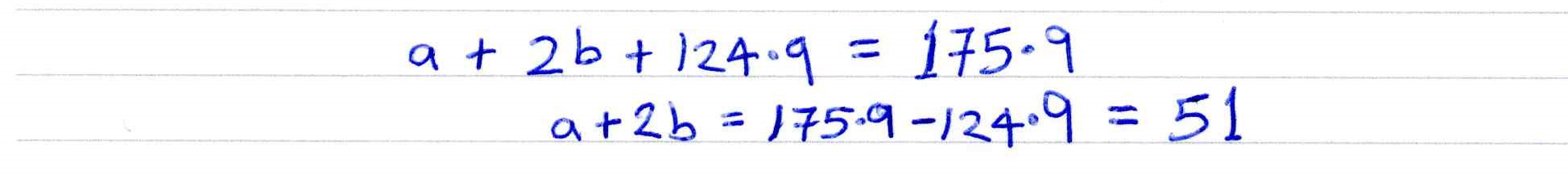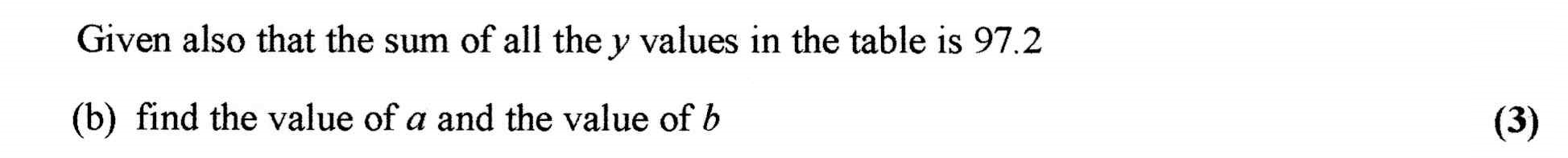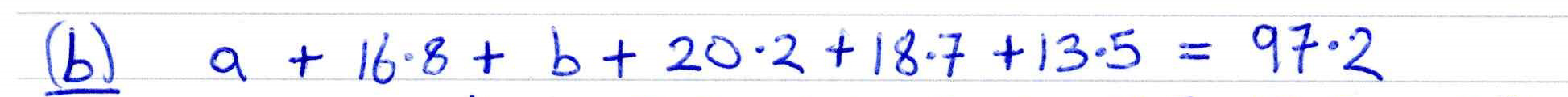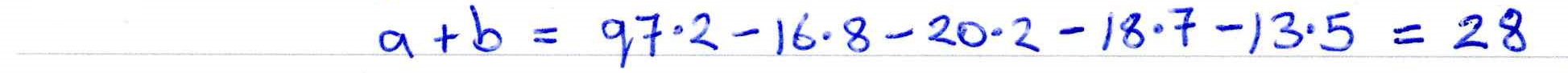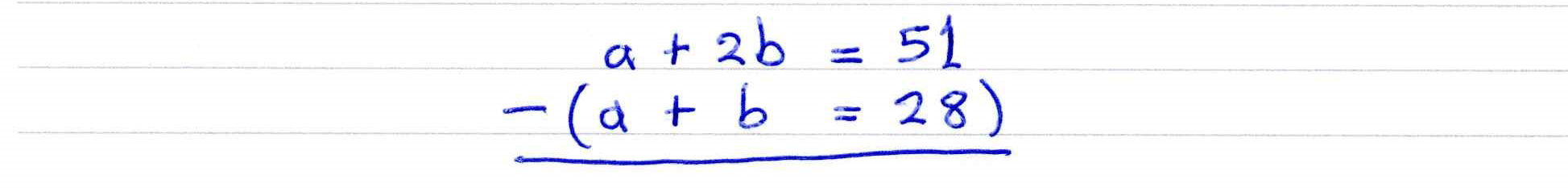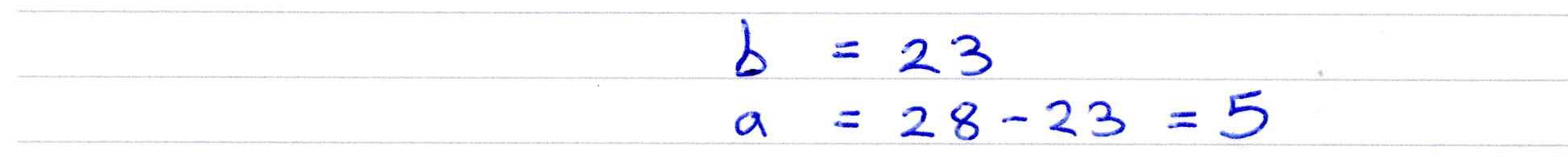##### Trapezium Rule Approximation for Difficult Integrals 1
The method of finding the area under a curve by splitting it up into strips is often referred to as a "Riemann sum". The value of a definite integral can be estimated using various numerical methods. This is particularly useful when the integral is difficult or impossible to integrate. The method consists of dividing the area into strips. The trapezium rule (or trapezoidal rule in the USA) is one such method. The area of each strip is estimated by taking it to be approximately a trapezium.

The trapezium method uses this formula: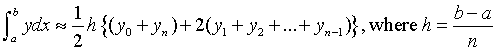With the display, you should see how the accuracy of the estimate increases as the number of intervals increases.
With the display, you should also see how the trapezium rule overestimates where the curve is concave upwards, and underestimates where the curve is convex upwards.
##### Trapezium Rule Approximation for Difficult Integrals 2
Functions such as $\color{blue}{y = {e^{ - {x^2}}}}$ and $\color{blue}{y = \sin \left( {x^2} \right)}$ cannot be integrated by basic methods.
However, their definite integrals can be estimated using numerical methods such as the trapezium rule (or trapezoidal rule in the USA) which is presented here.

The method consists of dividing the area into strips. The area of each strip is estimated by taking it to be approximately a trapezium.With the display, you should see how the accuracy of the estimate increases as the number of intervals increases.
With the display, you should also see how the trapezium rule overestimates where the curve is concave upwards, and underestimates where the curve is convex upwards.